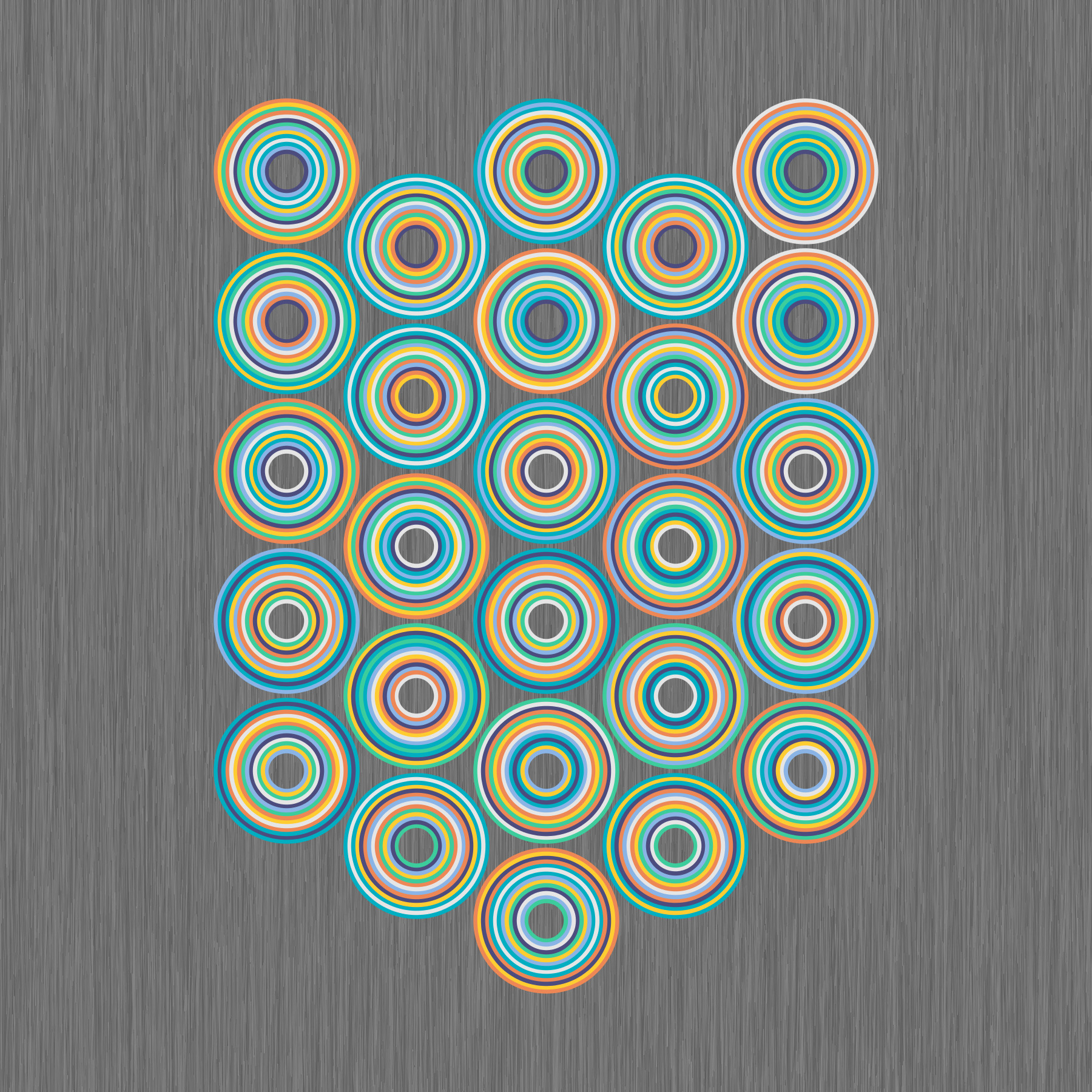# David Reimann

Professor of Mathematics and Computer Science
Albion College
Albion, Michigan, USA

Through my art I make visible the beauty and wonder I see in the often abstract world of mathematics. I enjoy giving visual representations to mathematical concepts such as number, form, and process. I often use patterns that convey messages at multiple levels and scales using a wide variety of mathematical elements and media. Some of my work contains fine detail that allows the art to be viewed differently depending on the distance between the viewer and the art. Another prevalent theme in my work is symmetry, where the overall pattern is created by repeated rotation or translation of similar units.Binomial Bits
50 x 50 cm
Digital Print
2021
All patterns of 10-bit binary patterns are shown as rows of dots with a one represented by a yellow dot and a zero represented with a red dot. The patterns are grouped into the 11 possibilities for the number of ones (or zeros) in a pattern. The number of rows in each group is the binomial coefficient corresponding to the number of ways to choose $k$ items from a set of 10 items, resulting in a binomial distribution. When $k$ is 0 or 10, there is only 1 possible pattern. When $k$ is 1 or 9, there are 10 possible patterns. For $k$ or $10-k$ equaling 2, 3, 4, and 5, the number of possible patterns are 45, 120, 210, and 252 respectively.Septenary Circles
50 x 50 cm
Digital Print
2021

This artwork answers the question of how many ways can one place seven color pairs such that there is one other color between the first color pair, two colors between the second color pair, and so on. Mathematically, these are the twenty-six different Langford sequences corresponding to 7 colors. These sequences were first described by C. Dudley Langford in 1958 and popularized by Martin Gardner in 1967.